#### Chapter 4 Algebraic Identities R.D. Sharma Solutions for Class 9th Math Exercise 4.1

Exercise 4.1

1. Evaluate each of the following using identities :
(i)  (2x-1/x)2(ii) (2x + y) (2x − y)
(iii) (a2b2a)2
(iv) (a - 0.1) (+ 0.1)
(v) (1.5x− 0.3y2) (1.5x+ 0.3y2)

Solution2. Evaluate each of the following using identities :
(i) (399)2
(ii) (0.98)2
(iii) 991 ☓ 1009
(iv) 117 ☓ 83

Solution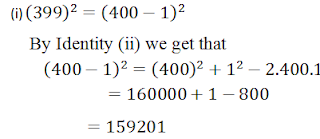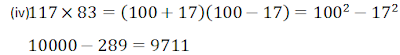3. Simplify each of the following :
(i) 175 × 175 + 2 × 175 × 25 + 25 × 25
(ii) 322 × 322 − 2 × 322 × 22 + 22 × 22
(iii) 0.76 × 0.76 + 2 × 0.76 × 0.24 + 0.24 × × 0.24
(iv) (7.83 ×7.83−1.17×1.17)/6.6

Solution4. If x+1x=11,find the value of x2+1x2

Solution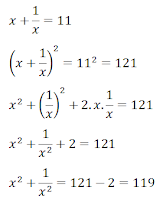5. If  x1x=1, find the value of x2+1x2

Solution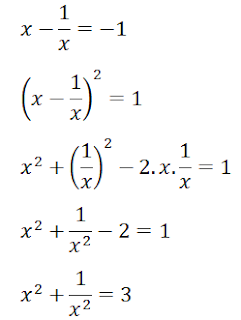6. If x+1x=5 ,find the value of x2+1x2.

Solution7. If x2+1x2=66find the value of x1x

Solution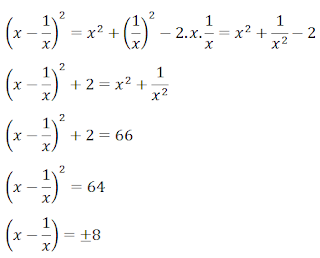8.  If x2+1x2=79, find the value of x+1x

Solution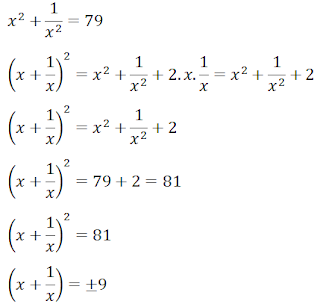9. If 9x+ 25y2 = 181 and xy = −6, find the value of 3x + 5y.

Solution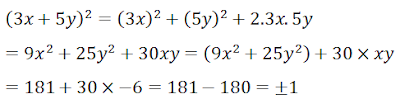10. If 2x + 3y = 8 and xy = 2, find the value of 4x2 + 9y2

Solution11. If 3x − 7y = 10 and xy = -1, find the value of 9x2 + 49y2

Solution12. Simplify each of the following products:

Solution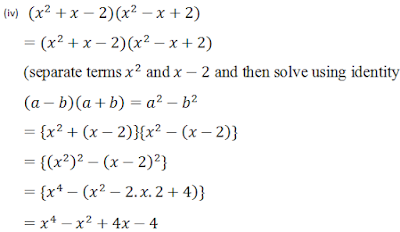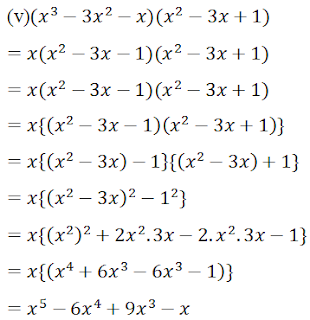13.  Prove that a2 + b2 + c2 −ab−bc−ca is always non-negative for all values of a, b, and c.

SolutionSquares of the numbers are always positive, therefore the number is always positive.